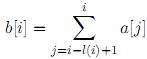favorite Saytın davamlılığını təmin etmək üçün sizin köməyinizə ehtiyacımız vardır, ətrafli məlumat üçün bannerə klikləyin
Məsələlər

# Fenwick Tree

Fenwick tree is a data structure effectively supporting prefix sum queries.

For a number t denote as h(t) maximal k such that t is divisible by 2k. For example, h(24) = 3, h(5) = 0. Let l(t) = 2h(t), for example, l(24) = 8, l(5) = 1.

Consider array a, a, ..., a[n] of integer numbers. Fenwick tree for this array is the array b, b, ..., b[n] such that.

So

b = a,

b = a + a,

b = a,

b = a + a + a + a,

b = a,

b = a + a,

...

For example, the Fenwick tree for the array

a = (3, -1, 4, 1,-5, 9)

is the array

b = (3, 2, 4, 7,-5, 4).

Let us call an array self-fenwick if it coincides with its Fenwick tree. For example, the array above is not self-fenwick, but the array a = (0,-1, 1, 1, 0, 9) is self-fenwick.

You are given an array a. You are allowed to change values of some elements without changing their order to get a new array a' which must be self-fenwick. Find the way to do it by changing as few elements as possible.

Input

The first line of the input file contains n — the number of elements in the array (1n100000). The second line contains n integer numbers — the elements of the array. The elements do not exceed 109 by their absolute values.

Output

Output n numbers — the elements of the array a'. If there are several solutions, output any one.

Zaman məhdudiyyəti 3 saniyə
Giriş verilənləri #1
```6
3 -1 4 1 -5 9
```
Çıxış verilənləri #1
```0
-1
1
1
0
9
```
Mənbə Northern Subregional Programming Contest 2008
Enchanted Mirror
Ground Works